Wang, W., Cao, Y., Sun, L., and Wu, M. (2021). "Effect of temperature on formaldehyde diffusion in cellulose amorphous region: A simulation study," BioResources 16(2), 3200-3213.

#### Abstract

A formaldehyde-cellulose amorphous region model at the micro-level was established using the molecular dynamics software Materials Studio to simulate the change of cellulose and formaldehyde molecules in an external temperature field. The diffusion coefficients of formaldehyde molecules increased as the temperature increased. Moreover, the total number of hydrogen bonds decreased, and the interaction energy in the formaldehyde-cellulose model was reduced, which confirmed this conclusion and indicated that temperature increase could enhance the diffusion of formaldehyde in cellulose. The mechanical parameters of cellulose were analyzed in terms of Young’s modulus, shear modulus, bulk modulus, Poisson’s ratio, and the ratio of bulk modulus to shear modulus (K/G), which were affected by the temperature. The elastic modulus (E, G, and K) of cellulose decreased as the temperature increased, while the Poisson’s ratio V and K/G values increased. The results of the research explain how elevated temperature can promote the release of formaldehyde in furniture from a microscopic perspective, which supports each other with the results of previous experimental data and practical applications in production.

Effect of Temperature on Formaldehyde Diffusion in Cellulose Amorphous Region: A Simulation Study

Wei Wang,* Yancai Cao, Liyue Sun, and Mingshuai Wu

A formaldehyde-cellulose amorphous region model at the micro-level was established using the molecular dynamics software Materials Studio to simulate the change of cellulose and formaldehyde molecules in an external temperature field. The diffusion coefficients of formaldehyde molecules increased as the temperature increased. Moreover, the total number of hydrogen bonds decreased, and the interaction energy in the formaldehyde-cellulose model was reduced, which confirmed this conclusion and indicated that temperature increase could enhance the diffusion of formaldehyde in cellulose. The mechanical parameters of cellulose were analyzed in terms of Young’s modulus, shear modulus, bulk modulus, Poisson’s ratio, and the ratio of bulk modulus to shear modulus (K/G), which were affected by the temperature. The elastic modulus (E, G, and K) of cellulose decreased as the temperature increased, while the Poisson’s ratio V and K/G values increased. The results of the research explain how elevated temperature can promote the release of formaldehyde in furniture from a microscopic perspective, which supports each other with the results of previous experimental data and practical applications in production.

Keywords: Formaldehyde; Temperature; Cellulose; Molecular dynamics; Mechanical properties

Contact information: College of Engineering and Technology, Northeast Forestry University, Harbin 150040, China; *Corresponding author: cedar@163.com

INTRODUCTION

The man-made boards used in wood-based furniture, especially the high-density board, are bound together by a large amount of glue, leading to relatively high formaldehyde concentration. As a major type of volatile organic compounds (VOCs), formaldehyde poses a considerable threat to human health given its low exposure limit and toxicity. Therefore, it is essential to lower the concentration of formaldehyde in wood-based furniture as much as possible.

Wood-based furniture is mainly made up of man-made boards, whose major component is wood. It is well known that wood in general consists largely of cellulose. In addition to this, it contains hemicellulose and lignin, water (especially at room temperature). To simplify the study, this paper only considers the diffusion of formaldehyde in pure cellulose at the theoretical level, without considering the influence of other components on the diffusion behavior. As a result, studying formaldehyde molecules in cellulose helps to understand important aspects of such diffusion in wood-based furniture at large.

Cellulose is a linear polymer linked via 1,4-β-D-glucoside linkages, consisting of crystalline and amorphous regions. Cellulose molecules in the crystalline regions are arranged in a highly ordered structure, exhibiting impressive mechanical properties at high temperatures (Khazraji and Robert 2013; Wang et al. 2018). In contrast, cellulose molecules in amorphous regions are disorganized and are more likely to be influenced by temperature. Due to the strong and highly ordered intermolecular forces in the crystalline regions, formaldehyde molecules, which can hardly diffuse into this region, only react on the surfaces of crystals. However, the weaker intermolecular force in the amorphous regions allows the formaldehyde molecules to diffuse. After comprehensive consideration, this study aims to discover the formaldehyde diffusion regularity within the amorphous regions of cellulose.

The macro experiment and theoretical model are the two researching methods commonly used by scholars at home and abroad in the studying of formaldehyde diffusion in building materials. Macro experimental research focuses on measuring the indoor emission rate of VOCs and three critical parameters (initial dischargeable concentration, diffusion coefficient, and partition coefficient). The results show that the critical parameters are affected by temperature. As temperature rises, the emission rate of formaldehyde increases (Lin et al. 2009; Crawford and Lungu 2011; Parthasarathy et al. 2011); both its initial dischargeable concentration and diffusion coefficient exhibit the same trend while the partition coefficient declines. An experiment by Huang et al. (2015) indicates that as the temperature rises from 25 ℃ to 80 ℃, the initial dischargeable concentration of formaldehyde rises by 14 times. Another macro experimental study (Xu and Chen 2017) examined the formaldehyde emission rate of building materials under different temperatures. With the formaldehyde emission rate measured at 298, 303, 308, and 313 K, it can be concluded that building materials release more formaldehyde as temperature increases. The theoretical model, which predicts emission rates based on a considerable number of experimental data (Haghighat and Bellis 1998; Guo 2002), is only viable under certain experimental conditions. It cannot be used in others for emission prediction. Therefore, it is essential and necessary to measure the diffusion coefficient of small gas molecules. However, an accurate result can hardly be obtained through experiments. In comparison, using molecular dynamics simulation, an important computer numerical simulation method not only can provide accurate diffusion coefficients, but also it can reveal molecular diffusion behavior from the microscopic level (Hou et al. 2001; Wang et al. 2020).

The molecular simulation method can be used to construct a model of the amorphous region and to study the diffusion of small gas molecules under different conditions. Based on Molecular Dynamics Simulation, a study involving cellulose Iβ was conducted to analyze its property variation with and without ammonia molecules in the environment as well as the diffusion of the ammonia molecules. Moreover, a model of water diffusive motion in oil and cellulose was built to analyze water diffusion in composite media of oil and water (Liao et al. 2011). In addition, molecular dynamics can simulate metallic materials or carbon nanotubes containing defects, so it is expected that boards containing defects or nonuniformities can be realized with molecular dynamics. From the above statements, it can be concluded that Molecular Dynamics Simulation is an ideal method to analyze the diffusion of micro-molecules. Although the object of the paper is general boards without defects, it is indispensable to analyze the diffusion behavior of formaldehyde molecules from the molecular microscopic point of view under the applied temperature field. The simulation experiment enables us to understand the principle of the change in the diffusion coefficient of formaldehyde from the molecular point of view

Previous research has shown that formaldehyde can diffuse faster in the external thermal environment, but the method presently remains at the experimental stage. The development of molecular simulation in recent years provides new insights into the diffusion of formaldehyde in furniture. This study examines the effect of temperature on the diffusion of formaldehyde on a micro-level. It also analyses how formaldehyde interacts with the amorphous region of cellulose and how the mechanical properties of cellulose change under the external thermal environment. The research will explain how elevated temperature can promote the release of formaldehyde in furniture from a microscopic perspective and supports each other with the results of previous experimental data to provide theoretical support for the principle of elevated temperature removal of volatile organic compounds such as formaldehyde in furniture.

EXPERIMENTAL

Methods

Modeling

Materials Studio (Accelrys, v.8.0, San Diego, CA, USA) was used to establish the amorphous region of cellulose-based on amorphous polymer construction (Theodorou and Suter 1985), and the Amorphous Cell module was used to construct the formaldehyde molecules model. No remarkable differences were found among the influences of different polymerization degrees on the physicochemical property in the amorphous region of cellulose (Mazeau and Heux 2008); thus, the cellulose chain with polymerization degree of 20 was built. The target density of this model was set as 1.4 g/cm3 (Brandrup et al. 1999) and the composite model included 20 formaldehyde molecules and a cellulose chain at polymerization degree of 20.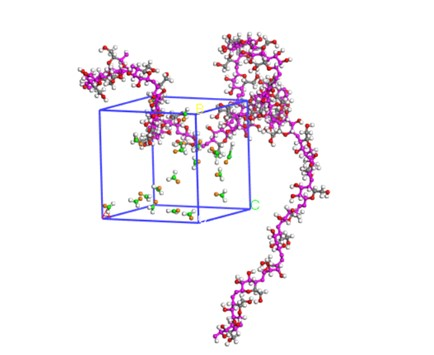Fig. 1. Formaldehyde-cellulose amorphous region model

Simulation details

After the model in Fig. 1 was established, the structure relaxation (namely annealing treatment) was carried out before model simulation. Firstly, the geometric structure was optimized through Calculation in the Forcite module to balance the free motion of molecules in a mixed system and to minimize the energy. Secondly, the initial dynamic relaxation was performed at 600 K in the macro canonical (NVT) ensemble. The initial velocity was random; the time step was 1 fs; the overall length of the simulation was 1 ns. One frame was output for every 5000 steps. After model relaxation, the initial internal stress decreased and the system tended to reach a stable state with lower energy. Thus, the subsequent simulation could be performed based on this model.

Dynamic simulation

During the simulation, the hybrid model of cellulose and small formaldehyde molecules involved the calculation of organic compounds. Therefore, the pcff force field suitable for the calculation was selected (Maple et al. 1988, 1994). Isothermal isobaric ensemble (NPT) was used for dynamics simulation. Pressure was controlled by the Berendsen method. The Ewald method and Atom based method (Ewald 1921) were adopted for the summation of electric charge and Van der Waals forces (VDW), respectively. In the simulation system, the initial velocity of atoms was designated by Boltzmann distribution randomly and the Velocity Verlet algorithm was used in integration. In these nine simulation systems, the simulation temperature range was set as 300 K to 460 K, with every 20 K as an interval, and the simulation time was 1000 ps. The atomic trajectory was collected every 5 ps to form a trajectory file.

RESULTS AND DISCUSSION

Balance of the System

In the molecular dynamics simulation, the temperature and energy fluctuated with the passage of time. The fluctuation can be used to determine whether the system was equilibrated (Wang et al. 2018). Therefore, the simulation of 1 ns was performed to obtain the diagram of how the energy and temperature changed with the time at 320 K, as shown in Figs. 2 and 3.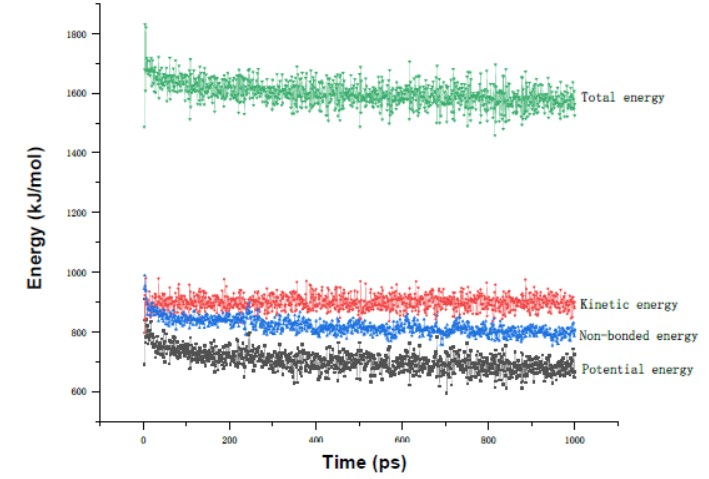Fig. 2. Energy-time variation chart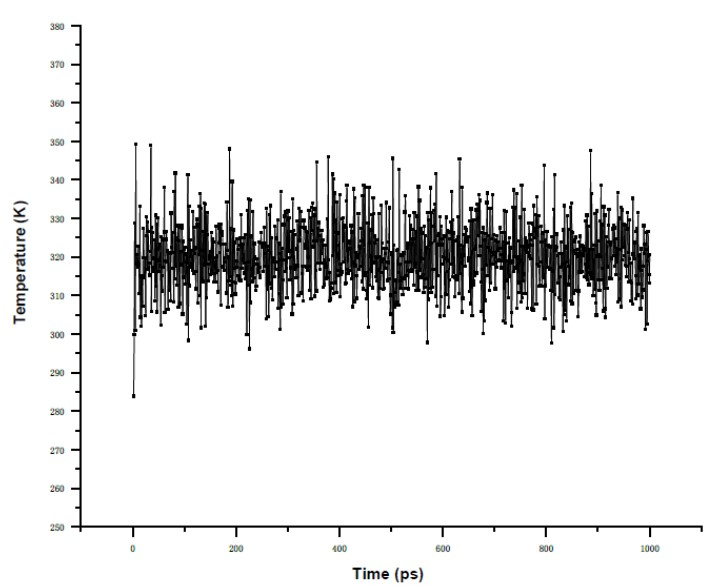Fig. 3. Temperature-time variation chart

The initial energy of the system is the result of the relaxation of the system, which can only reach the local optimum. After kinetic equilibrium, the system will gradually reduce the energy from the local optimum to reach stability, i.e., dynamic equilibrium. As a result, the energy gradually decreases with time until it stabilizes, as shown in Fig. 2. According to Figs. 2 and 3, there were no remarkable changes in energy and temperature during the simulation process and the temperature changed slightly (within the range of ± 30 K) over time. This meant that equilibrium was achieved in the system.

To verify the accuracy of the simulated system, the energy convergence parameter was used to determine the accuracy, defined as follows in Eq. 1,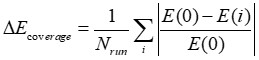(1)

where E(0) represents the initial total energy, E(i) represents the total energy of dynamics simulation during Step I, and Nrun represents the number of the simulation. If 0.001 < ∆E < 0.003, the simulation results would be reliable.

The calculation showed that ∆E = 0.0028, which proved the convergence and equilibrium of energy. This means the system has become stable, and interaction energy analysis, etc., can be conducted.

Diffusion Coefficient of Formaldehyde Molecules

Mean square displacement (MSD) refers to the mean sum of the square for the increment of the position vector of gas molecules in the microsystem within simulation time. The MSD can describe the centroid motion of formaldehyde molecules in the microsystem, which is helpful to research the diffusion change of the molecules. The expression is shown below,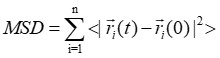(2)

where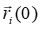and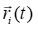refer to the position vectors of molecules at 0 and t, respectively, < > refers to ensemble average, and n refers to the number of diffused particles. Molecular diffusion coefficient D generally describes the behavior of molecular diffusion, indicating the diffusivity of substances, as shown in Eq. 3: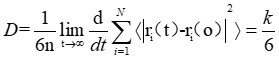(3)

In this expression, n represents the number of diffused particles in the system and k represents the slope of the MSD curve.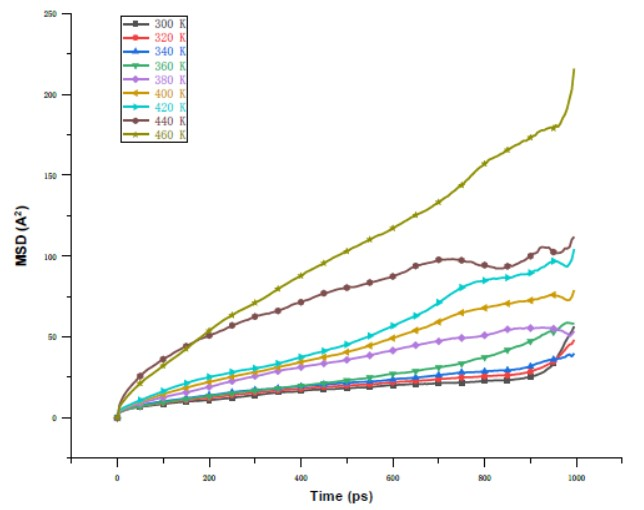Fig. 4. MSD curves of formaldehyde molecules in the amorphous region of cellulose under different temperatures

The MSD of formaldehyde molecules at different temperatures was obtained by analyzing the formaldehyde-cellulose amorphous mixed model, as shown in Fig. 4. The values of D (the diffusion coefficient for formaldehyde molecules) and k were obtained through the linear fitting of MSD curves resulted from the simulation process, as shown in Table 1.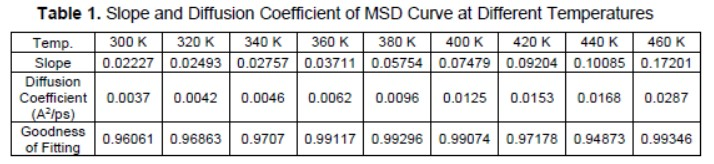As shown in Table 1, the goodness of fit was above 0.9, which means that the k value obtained was reliable and the coefficient D obtained could be used for subsequent analysis. According to Fig. 4 and Table 1, the higher the temperature, the larger the diffusion coefficient of formaldehyde molecules in the amorphous region of cellulose. When the temperature increased from 300 to 460 K, the diffusion coefficient D increased from 0.0037 to 0.0287, an increase of 7.76 times. This is because, on the one hand, the activity of molecules and atoms increases with the temperature, which enhances the motion intensity and thus increases the diffusion rate. On the other hand, the higher the temperature, the larger the energy of molecules and atoms. In this way, they can cross the energy barrier between formaldehyde molecules and cellulose more easily and thus diffuse into the air. As a result, the diffusion coefficient is increased.

Microscale is different from macroscale, and macro experiments are conducted in a closed environmental chamber. Thus, there is a difference between the diffusion coefficient of simulation and that of the experiment. Thus, only qualitative analysis is applicable. The simulation results in this paper showed that the diffusion coefficient increased as the temperature increased, which was consistent with the experimental results of Xu and Chen (2017).

Interaction Energy between Formaldehyde Molecules and Cellulose

The calculating formula of the interaction energy between two substances is Eq. 4:

EInter = ETotal – (EA + EB) (4)

where EInter, ETotal, EA, and EB represent total interaction energy (Kcal*mol-1), total potential energy (Kcal*mol-1), and potential energy (Kcal*mol-1) of substances A and B, respectively.

The Dreiding force field is used for calculation, and the bonding energy can be eliminated in the process of calculating the interaction energy, therefore Eq. 5 is as follows,

EInter = Evdw + Eelec + EHbond (5)

where Evdw, Eelec, and EHbond in Eq. 5 represent Van der Waals interaction energy (Kcal*mol-1), electrostatic interaction energy (Kcal*mol-1), and hydrogen bonding interaction energy (Kcal*mol-1), respectively.

The positive and negative values of the interaction energy indicate that there is a repulsive or binding effect between two substances, and the greater the absolute value, the stronger the interaction (Hanus and Mazeau 2010). The interaction energies of the formaldehyde-cellulose amorphous system at different temperatures during the simulation process were obtained by compiling the program, as shown in Table 2.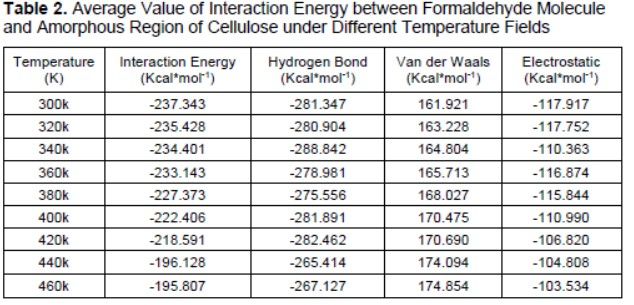Table 2 shows the average values of the interactions of formaldehyde molecules with the cellulose amorphous region under different temperature fields. Hydrogen bonding energy, Van der Waals interaction energy, and electrostatic interaction energy are the main interaction energies of the formaldehyde-cellulose amorphous system. According to the positive and negative analysis of the interaction energy in Table 2, the hydrogen bonding energy is negative, which means that formaldehyde molecules and cellulose are combined, i.e., hydrogen bonds are formed; the van der Waals interaction is positive, which means that the distance between formaldehyde molecule and cellulose molecule is close due to diffusion movement. Thus, the intermolecular repulsion force appears; the total interaction energy is negative. This indicates that cellulose and formaldehyde are attracted to each other.

The analysis of the magnitude of the interaction energy shows that when the temperature increased from 300 K to 460 K, both the hydrogen bonding and electrostatic interactions gradually decreased, which was consistent with the law of the decrease in the number of hydrogen bonds in the formaldehyde-cellulose system analyzed later.

Through compiling the program, the variation diagram of the total interaction energy of the formaldehyde-cellulose amorphous system with time under the action of different temperatures during the simulation process was obtained (Fig. 5). The total interaction energy decreased with the increase of temperature. The absolute value decreased 17.49% from 237.3 kcal/mol to 195.8 kcal/mol. This phenomenon indicates that to a certain extent, warming will reduce the binding of cellulose and formaldehyde molecules and enhance the diffusion behavior of formaldehyde.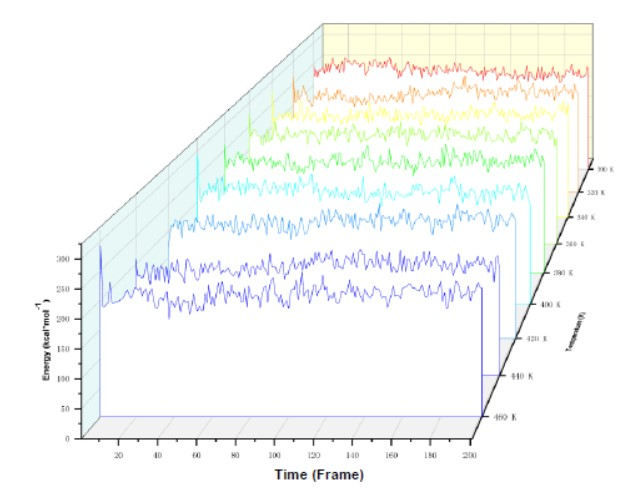Fig. 5. A graph of the total interaction energy between formaldehyde molecules and the amorphous region of cellulose under the action of temperature field

Hydrogen Bond

The hydrogen bond is a type of non-covalent bonding interaction, which is generally understood as a weak Coulomb interaction formed between an electronegative atom covalently bonded to a hydrogen atom and another more electronegative atom. Because there are free hydroxyl groups in cellulose, it is easy to form hydrogen bonds with polar formaldehyde molecules, so the study of hydrogen bonds between formaldehyde molecules and cellulose is important to observe the diffusion motion of formaldehyde molecules in cellulose pairs. During the simulation, the geometry of the hydrogen bond is specified as follows: the distance between the hydrogen atom and the acceptor is less than 2Å and the angle formed by the donor, hydrogen atom and acceptor is more than 110°. The method of counting the number of hydrogen bonds is to count all the hydrogen bonds within the model using the Calculating Hydrogen Bonds module that comes with the Material Studio software, and the number of hydrogen bonds between formaldehyde molecules and cellulose is counted by a self-compiled program.

The effect of different temperatures on the number of hydrogen bonds in the simulated system of the formaldehyde-cellulose amorphous region can be seen in Fig. 6, where the total number of hydrogen bonds in the system decreased 10.96% from 146 to 130 when the simulation temperatures were 300 K and 460 K, respectively. This simulation result corresponds to the previous results of the changes in the hydrogen bond energy within the cellulose amorphous region, all of which gradually decreased.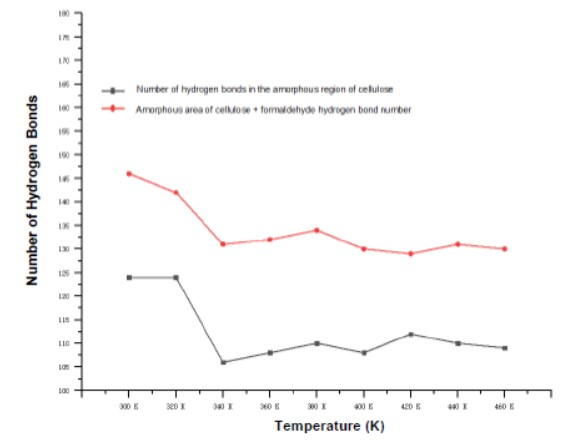Fig. 6. The number of hydrogen bonds with or without formaldehyde molecules in the amorphous region of cellulose at various temperatures

The decrease of the number of hydrogen bonds was due to the increase of temperature. Higher temperature aggravates the activity of cellulose molecular chains, which leads to an increase in the distance between molecular chains and a decrease in the number of hydrogen bonds. Moreover, the intensified movement of formaldehyde molecules with increasing temperature resulted in the wide diffusion of formaldehyde molecules, which reduced the total number of hydrogen bonds between the formaldehyde molecules and cellulose in the system.

Mechanical Parameter

Through calculating the dynamic trajectory file of the model at various temperatures, the elastic coefficient matrix can be obtained, in which the mechanical parameters can represent the mechanical properties of the man-made boards. The amorphous region of cellulose is isotropic, and the parallelepiped simulation system whose side length is divided into a, b, and c can use the Reuss average method to obtain the effective isotropic parameters,

K = (3(a + 2b))−1 (6)

G = 5 /(4a − 4b + 3c) (7)

where K and G are the bulk modulus (GPa) and shear modulus (GPa), respectively; a, b, and c are as follows,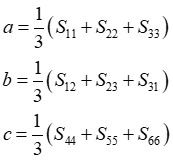(8)

where Sij is the inverse matrix of the elastic coefficient matrix. The Poisson’s ratio (v) and Young’s modulus (E) can be obtained by Eq. 9: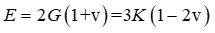(9)

Through molecular dynamics simulations, the variation of mechanical parameters of the cellulose amorphous region at different temperatures was calculated, as shown in Table 3.

The elastic modulus is the amount of elastic deformation resistance of the material and is an indicator of the stiffness of the material. According to the different forced conditions, there are corresponding Young’s modulus (E), shear modulus (G), and bulk modulus (K). The greater the modulus of elasticity is, the greater the resistance to elastic deformation is, and the less likely the material is to deform. The Poisson’s ratio (v) is also known as the coefficient of lateral deformation, which reflects the elastic coefficient of the material’s transverse deformation, with a positive relationship between the plasticity of the material. The K/G value (the ratio of bulk modulus and shear modulus) represents the toughness and ductility of the material, with a positive relationship between the toughness of the material.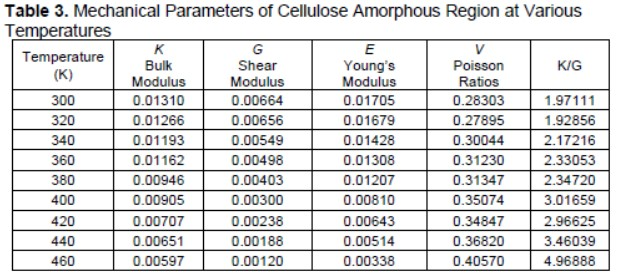The regularity drawn from the data in Table 3 and Fig. 7 shows that the elastic modulus (E, G, and K) of cellulose gradually decreases with the increase of temperature within the simulation temperature range, while the Poisson’s ratio V and K/G values gradually increase. This shows that the increase in temperature decreases the rigidity of the material and enhances its toughness and plasticity, i.e., the increase in temperature decreases the deformation resistance of the amorphous region.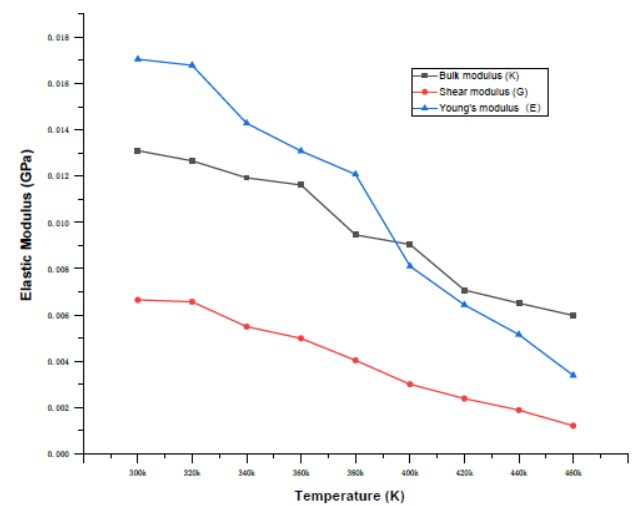Fig. 7. The change of elastic modulus with temperature

CONCLUSIONS

In this paper, a formaldehyde-cellulose model was established using the molecular simulation software Materials Studio to study the diffusion behavior of formaldehyde molecules, the changes in the number of hydrogen bonds, the interaction energy between formaldehyde and cellulose, and the mechanical properties of cellulose in the temperature range of 300 K to 460 K, after summarizing, the following conclusions can be drawn:

1. In the temperature range of 300 K to 460 K, a higher temperature resulted in a larger diffusion coefficient of formaldehyde molecules, and with the increase of temperature, the number of hydrogen bonds in the simulation system will gradually decrease, which also confirmed the enhancement of diffusion motion from the side. Moreover, the total interaction energy decreases with increasing temperature, which means that the binding of cellulose to the formaldehyde molecules is alleviated and the diffusion motion is enhanced. Overall, the increase in diffusion coefficient, the reduction in the number of hydrogen bonds, and the decrease in the interaction energy with increasing temperature, mutually reflect the enhancement of the diffusion capacity of formaldehyde.
2. The mechanical parameters of the amorphous region of cellulose were calculated in the temperature range of 300 K to 460 K, and the results show that the increase in temperature makes the modulus of elasticity (E, G, and K) gradually decrease, reducing the rigidity of cellulose and enhancing its ductility.

ACKNOWLEDGMENTS

The authors are grateful for the support by The Fundamental Research Funds for the Central Universities (41419020) and the Science Fund Project for Studying Abroad in Heilongjiang Province (LC201407).

REFERENCES CITED

Brandrup, J., Immergut, E. H., and Grulk, E. A. (1999). Polymer Handbook, John Wiley & Sons, Inc., Hoboken, NJ, USA.

Crawford, S., and Lungu, C. T. (2011). “Influence of temperature on styrene emission from a vinyl ester resin thermoset composite material,” Science of The Total Environment 409(18), 3403-3408. DOI: 10.1016/j.scitotenv.2011.05.042

Ewald, P. P. (1921). “Evaluation of optical and electrostatic lattice potentials,” Annals of Physics 369(3), 253-287.

Guo, Z. (2002). “Review of indoor emission source models. Part 1: Overview,” Environmental Pollution 120(3), 533-549. DOI: 10.1016/S0269-7491(02)00187-2

Haghighat, F., and Bellis, L. D. (1998). “Material emission rates: Literature review and the impact of indoor air temperature and relative humidity,” Building and Environment 33(5), 261-277. DOI: 10.1016/S0360-1323(97)00060-7

Hanus, J., and Mazeau, K. (2010). “The xyloglucan-cellulose assembly at the atomic scale,” Biopolymers 82(1), 59-73. DOI: 10.1002/bip.20460

Hou, T. J., Zhang, W., and Xu, X. J. (2001). “Binding affinities for a series of selective inhibitors of gelatinase-a using molecular dynamics with a linear interaction energy approach,” Journal of Physical Chemistry B 105(22), 5304-5315. DOI: 10.1021/jp0044476

Huang, S. D., Xiong, J. Y., and Zhang, Y. P. (2015). “Impact of temperature on the ratio of initial emittable concentration to total concentration for formaldehyde in building materials: Theoretical correlation and validation,” Journal of Environmental Science and Technology 49, 1537−1544. DOI: 10.1021/es5051875

Khazraji, A. C., and Robert, S. (2013). “Interaction effects between cellulose and water in nanocrystalline and amorphous regions: A novel approach using molecular modeling,” Journal of Nanomaterials 2013(3), Article ID 409676. DOI: 10.1155/2013/409676

Lin, C. C., Yu, K. P., and Zhao, P. (2009). “Evaluation of impact factors on VOC emissions and concentrations from wooden flooring based on chamber tests,” Building and Environment 44(3), 525−533. DOI: 10.1016/j.buildenv.2008.04.015

Maple, J. R., Dinur, U., and Haglaer, A. T. (1988). “Derivation of forcefields for molecular mechanics and dynamics from ab initio energy surfaces,” Proceedings of the National Academy of Sciences of the United States of America 85(15), 5350-5354. DOI: 10.1073/pnas.85.15.5350

Maple, J. R., Hwang, M. J., Stockfisch, T. P., Dinur, U., Waldman, M., Ewig, C. S., and Hagler, A. T. (1994). “Derivation of class II force fields. I. Methodology and quantum force field for the alkyl functional group and alkane molecules,” Journal of Computational Chemistry 15(2), 162-182. DOI: 10.1002/jcc.540150207

Mazeau, K., and Heux, L. (2008). “Molecular dynamics simulations of bulk native crystalline and amorphous structures of cellulose,” Journal of Physical Chemistry B 107(10), 2394-2403. DOI: 10.1021/jp0219395

Parthasarathy, S., Maddalena, R. L., Russell, M. L., and Apte, M. G. (2011). “Effect of temperature and humidity on formaldehyde emissions in temporary housing units,” Journal of the Air & Waste Management Association 61(6), 689−695. DOI: 10.3155/1047-3289.61.6.689

Theodorou, D. N., and Suter, U. W. (1985). “Detailed molecular structure of a vinyl polymer glass,” Macromolecules 18(7), 1467-1478. DOI: 10.1021/ma00149a018

Wang, W., Sun, L., Wu, M., Li, X., and Song, W. (2020). “Molecular dynamics simulation of bamboo heat treatment with cellulose based on molecular different weight fractions of water,” BioResources 15(3), 6766-6780. DOI: 10.15376/biores.15.3.6766-6780

Wang, W., Wang, Y., and Li, X. (2018). “Molecular dynamics study on mechanical properties of cellulose with air/nitrogen diffusion behavior,” BioResources 13(4), 7900-7910. DOI: 10.15376/biores.13.4.7900-7910

Xu, B., and Chen, Z. Q. (2017). “The combined effects of temperature and electric fields on formaldehyde emission from building materials: Experiment and molecular dynamics simulation,” International Communications in Heat and Mass Transfer 87, 105-111. DOI: 10.1016/j.icheatmasstransfer.2017.06.006

Article submitted: September 4, 2020; Peer review completed: December 5, 2020; Revised version received and accepted: March 9, 2021; Published: March 11, 2021.

DOI: 10.15376/biores.16.2.3200-3213